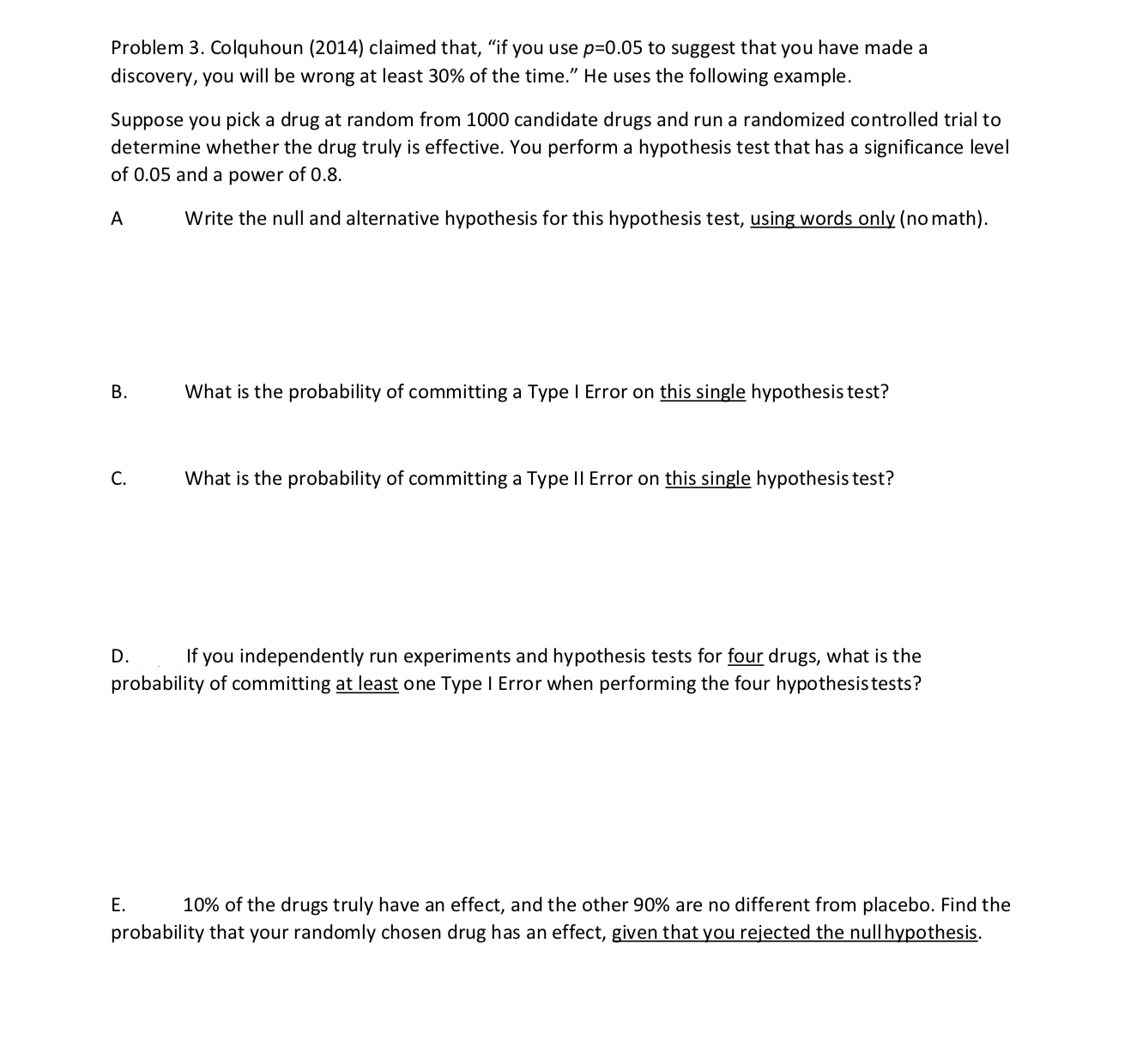Problem 3, Colquhoun (2014) claimed that, "if you use p=0.05 to suggest that you have made adiscovery, you will be wrong at least 30% of the time." He uses the following example.Suppose you pick a drug at random from 1000 candidate drugs and run a randomized controlled trial todetermine whether the drug truly is effective. You perform a hypothesis test that has a significance levelof 0.05 and a power of 0.8.A Write the null and alternative hypothesis for this hypothesis test, using words only (no math).BWhat is the probability of committing a Type l Error on this single hypothesis test?C. hat is the probability of committing a Type Il Error on this single hypothesis test?D. If you independently run experiments and hypothesis tests for four drugs, what is theprobability of committing at least one Type l Error when performing the four hypothesistests?E. 10% of the drugs truly have an effect, and the other 90% are no different from placebo. Find theprobability that your randomly chosen drug has an effect, given that you rejected the nullhypothesis

Questionhelp_outlineImage TranscriptioncloseProblem 3, Colquhoun (2014) claimed that, "if you use p=0.05 to suggest that you have made a discovery, you will be wrong at least 30% of the time." He uses the following example. Suppose you pick a drug at random from 1000 candidate drugs and run a randomized controlled trial to determine whether the drug truly is effective. You perform a hypothesis test that has a significance level of 0.05 and a power of 0.8. A Write the null and alternative hypothesis for this hypothesis test, using words only (no math). B What is the probability of committing a Type l Error on this single hypothesis test? C. hat is the probability of committing a Type Il Error on this single hypothesis test? D. If you independently run experiments and hypothesis tests for four drugs, what is the probability of committing at least one Type l Error when performing the four hypothesistests? E. 10% of the drugs truly have an effect, and the other 90% are no different from placebo. Find the probability that your randomly chosen drug has an effect, given that you rejected the nullhypothesis fullscreen
Step 1

Hello. Since your question has multiple sub-parts, we will solve the first three sub-parts for you. If you want remaining sub-parts to be solved, then please resubmit the whole question and specify those sub-parts you want us to solve.

A.

The null and alternative hypothesis of the test are:

Null hypothesis (Ho) : The drug has no effect.

Alternative hypothesis (H1) : The drug is effective.

Step 2

B.

The significance level is the probability of committing type I error.

In the above case, significance level is 0.05.

...

Want to see the full answer?

See Solution

Want to see this answer and more?

Our solutions are written by experts, many with advanced degrees, and available 24/7

See Solution
Tagged in

Hypothesis Testing